# RD Sharma Solutions for Class 6 Maths Chapter 6: Fractions Objective Type Questions

This Chapter explains topics like Fractions which are important from the exam point of view. We know that a fraction represents a part of a whole either single object or a group of objects. The students can have a better knowledge about the exercise wise problems in this Chapter by solving problems using RD Sharma Solutions for Class 6 as a study material. To ace the exam, students can download the RD Sharma Solutions for Class 6 Maths Chapter 6 Fractions Objective Type Questions PDF provided here which acts as a major reference guide.

## RD Sharma Solutions for Class 6 Maths Chapter 6: Fractions Objective Type Questions Download PDF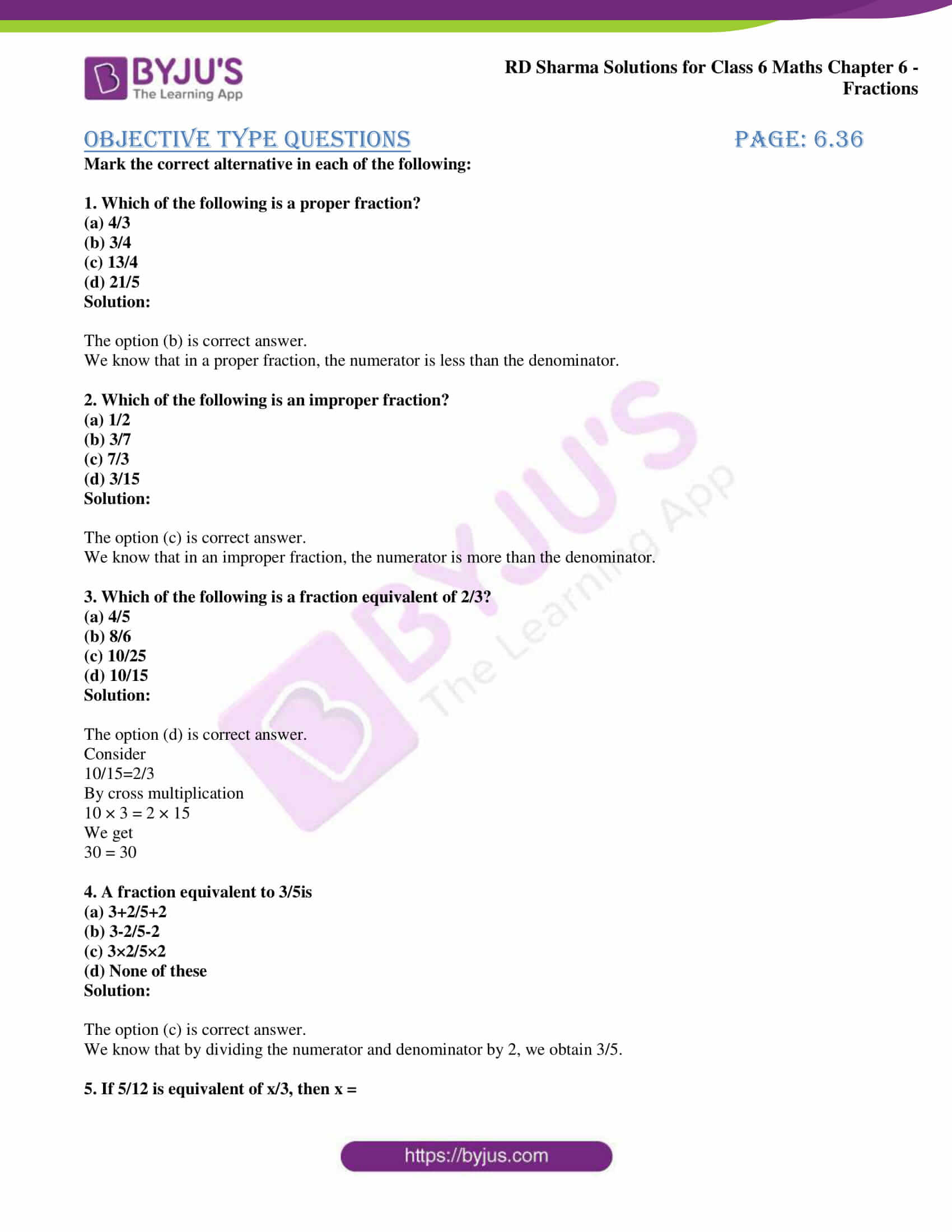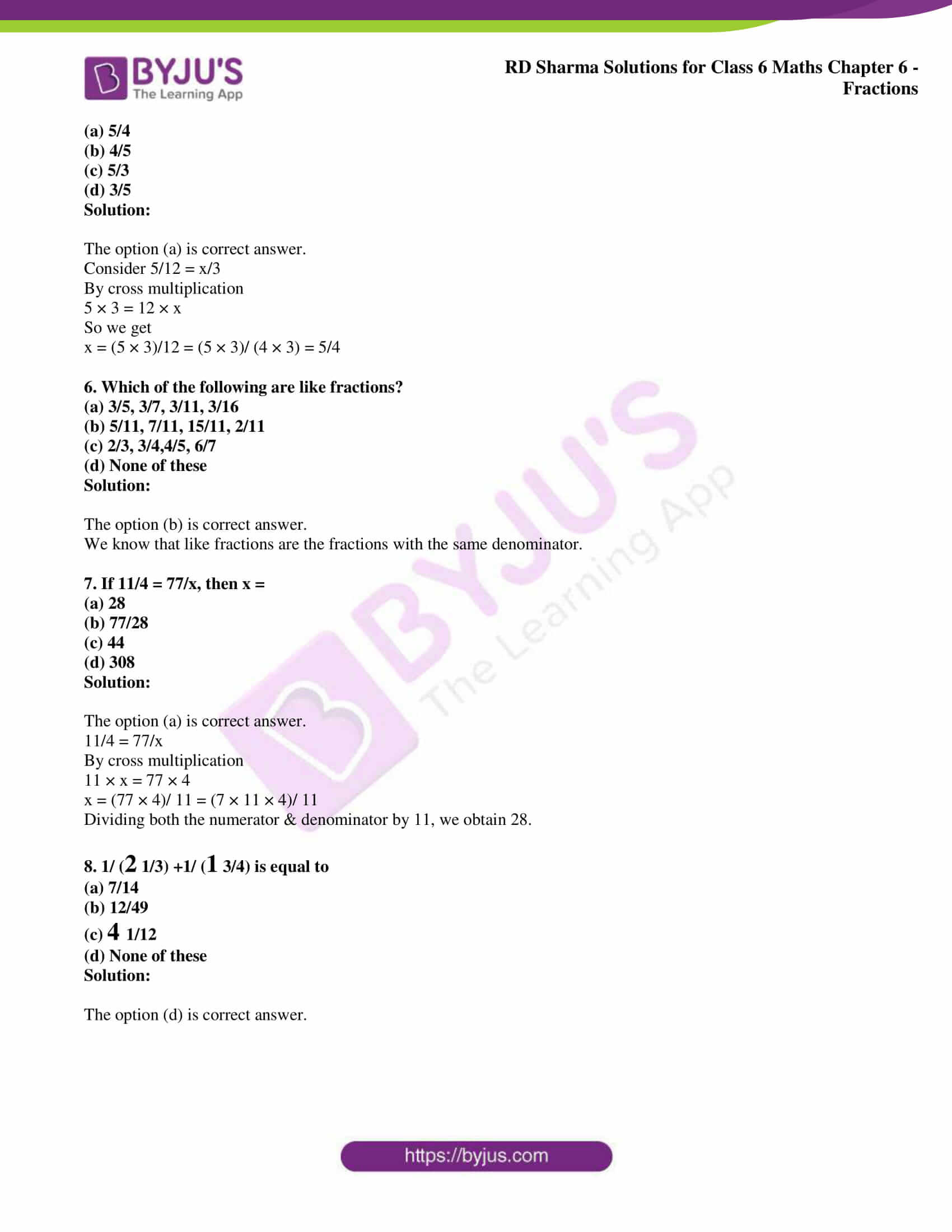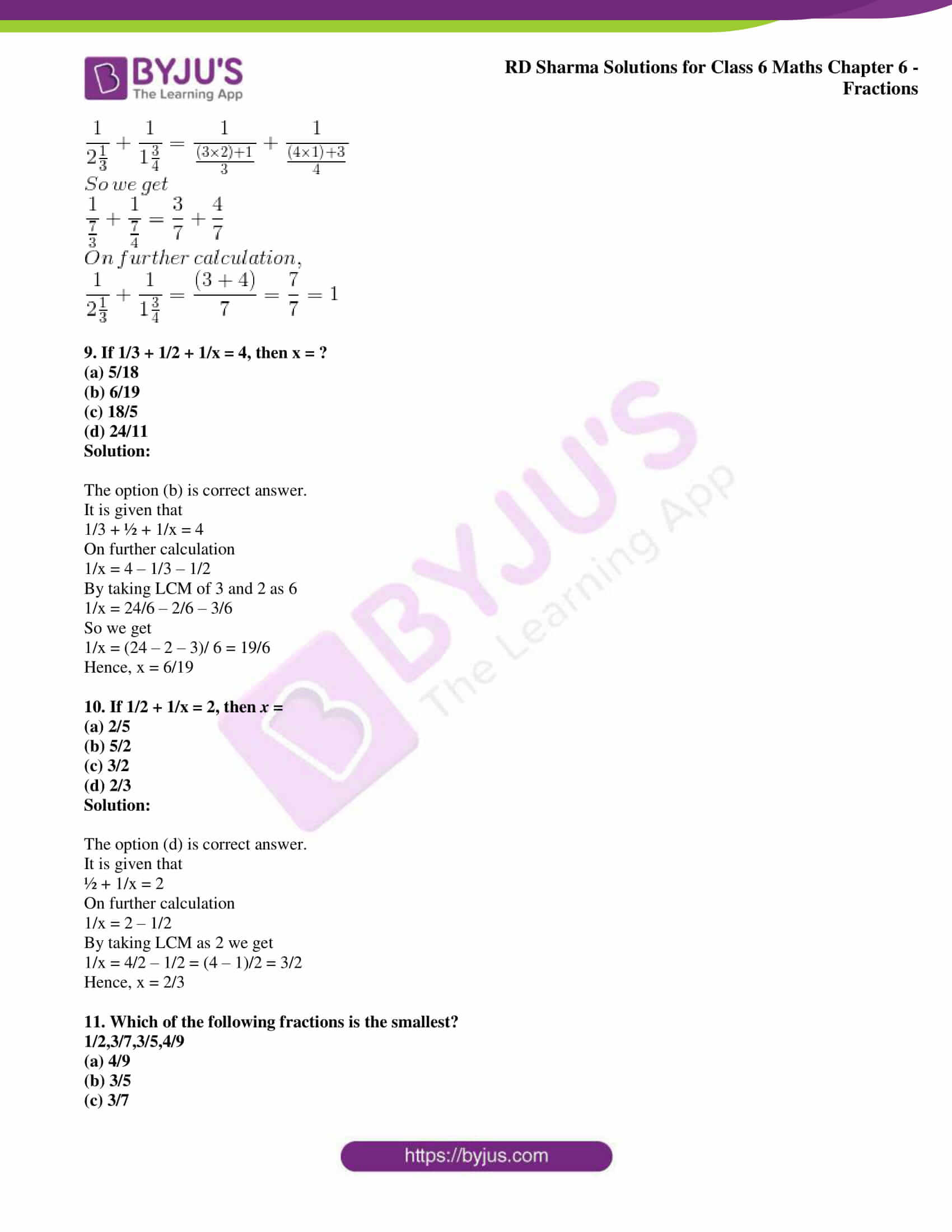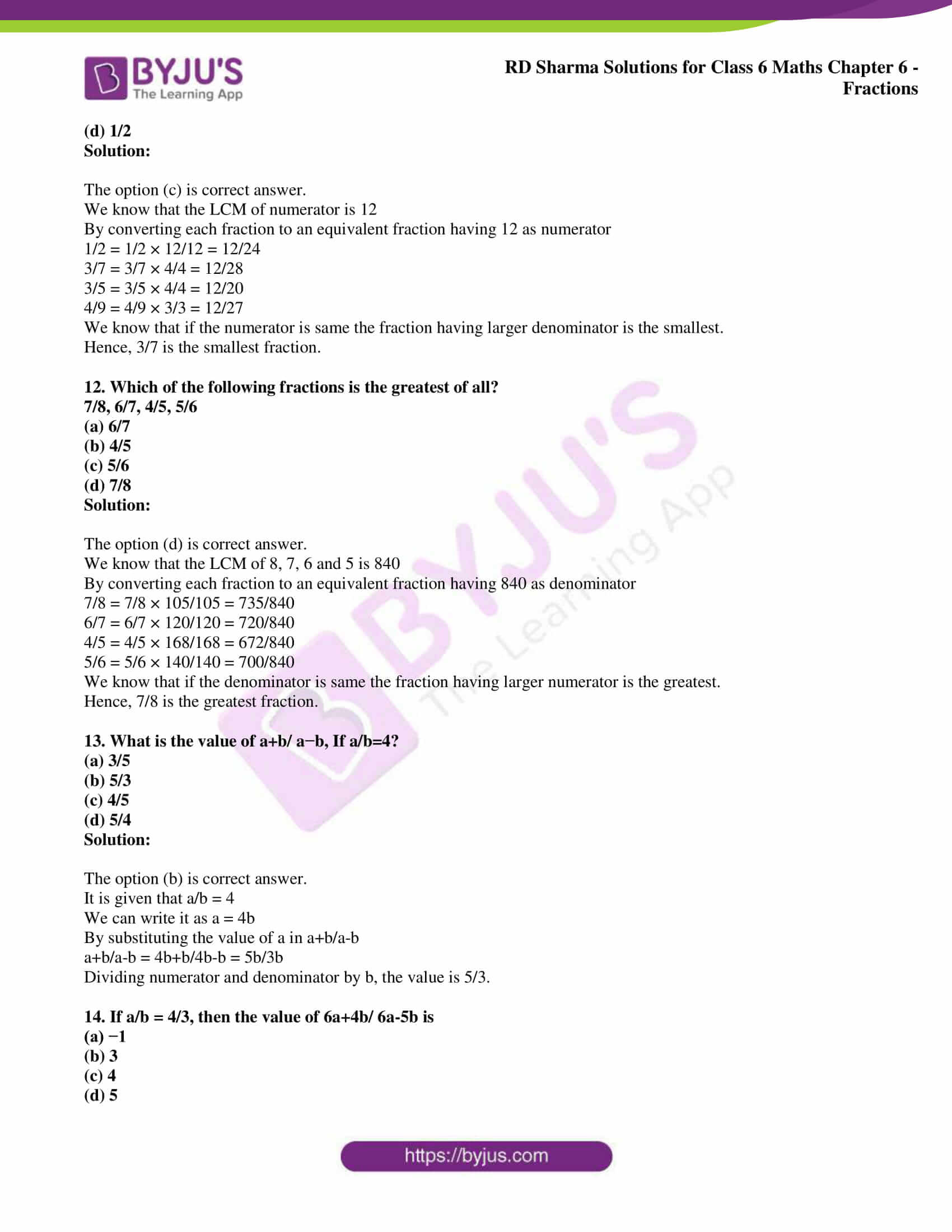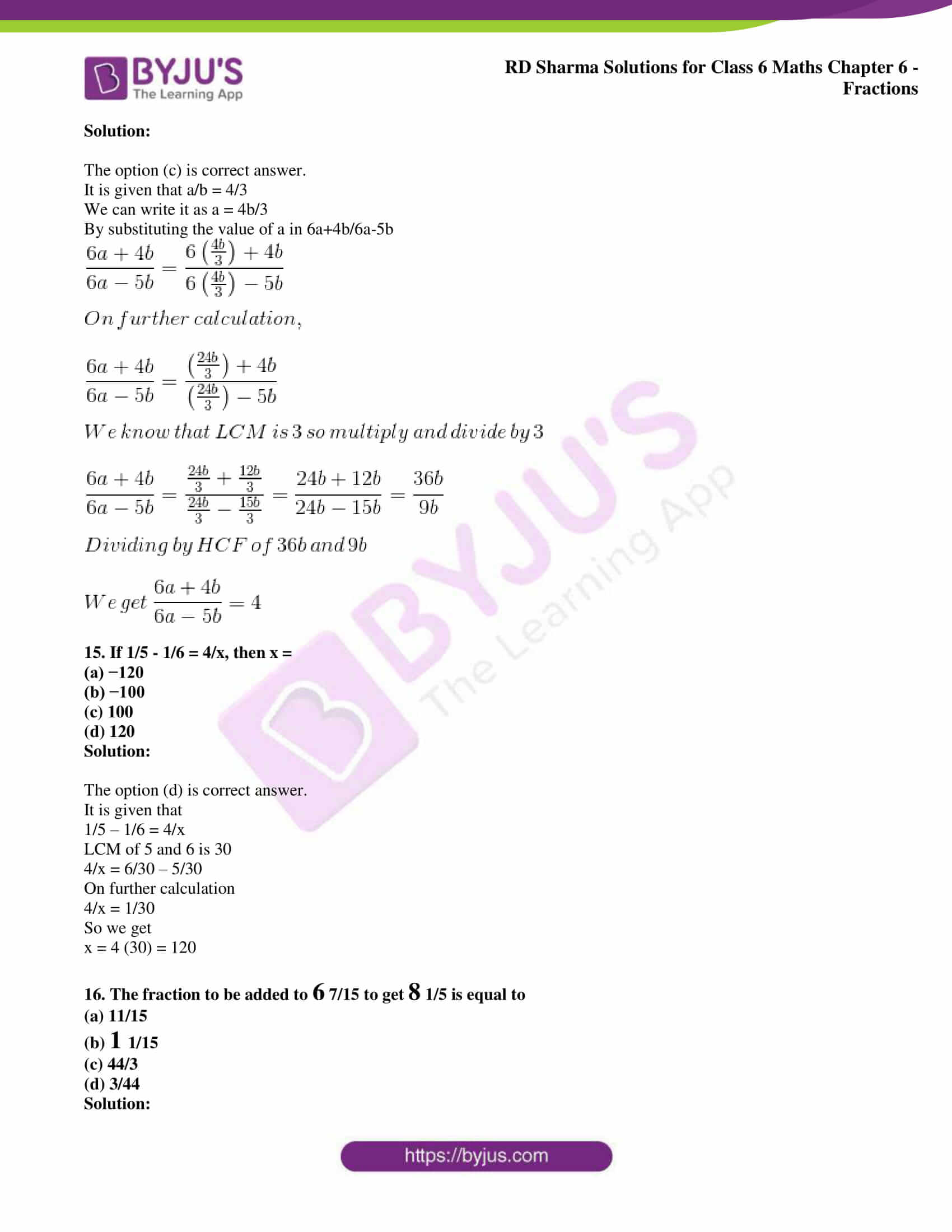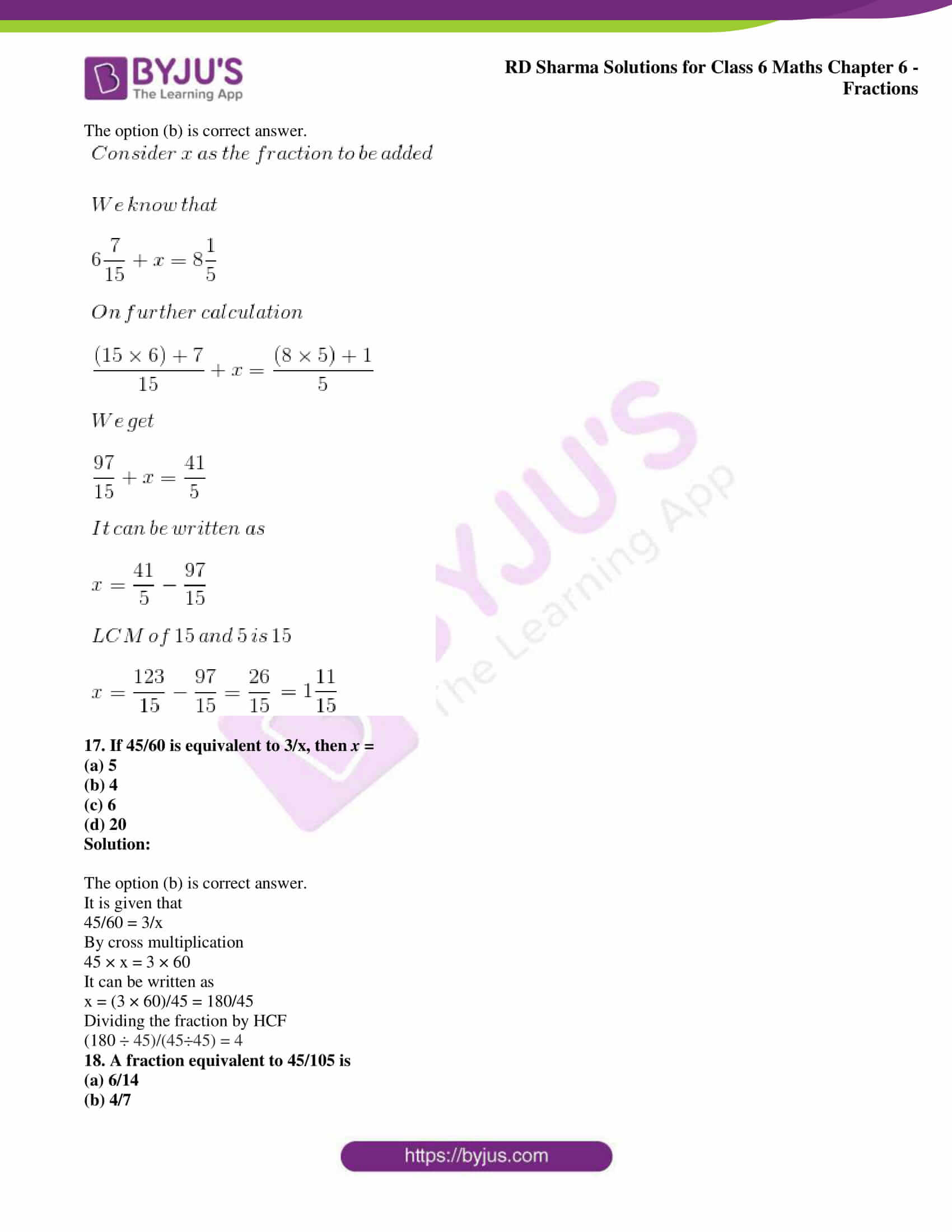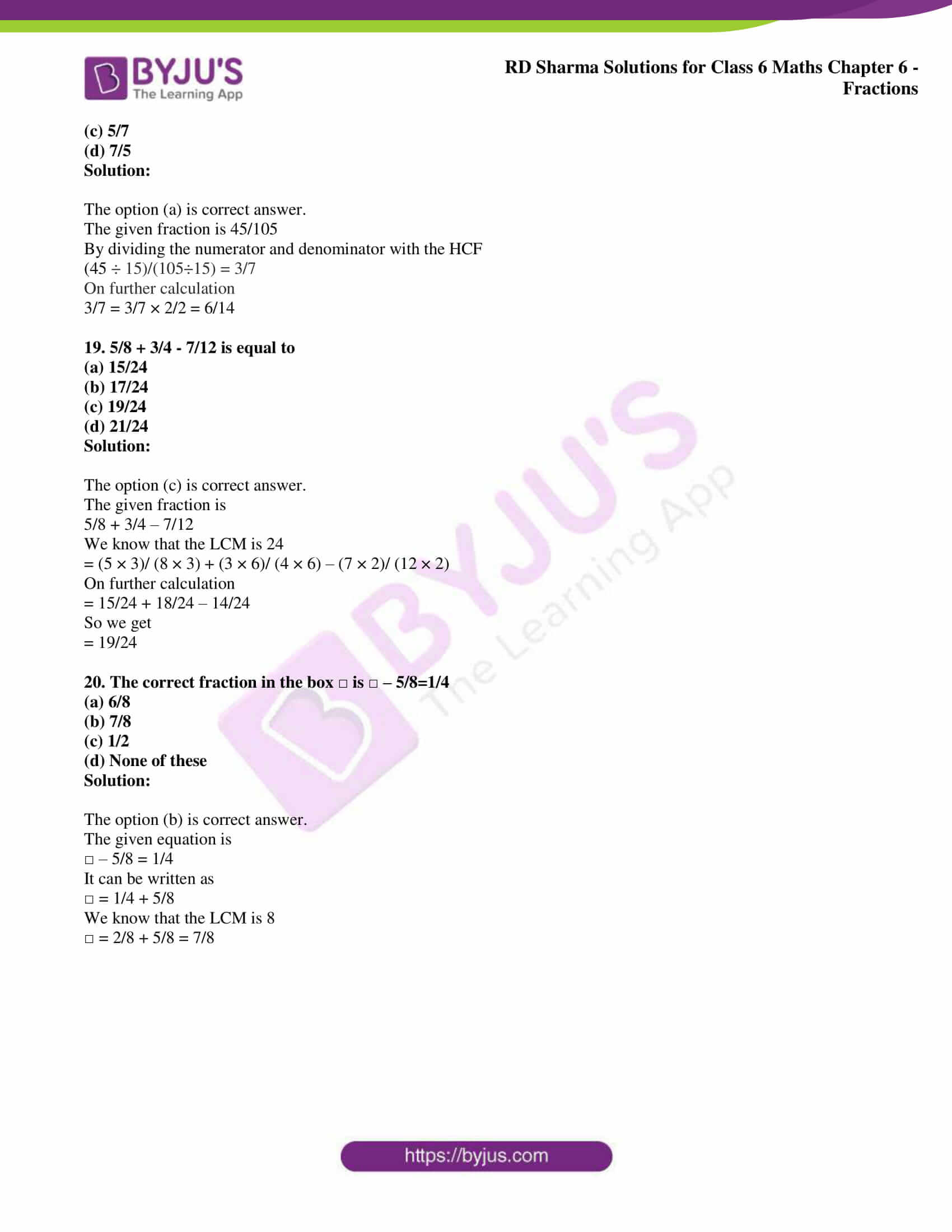### Access answers to Maths RD Sharma Solutions for Class 6 Chapter 6: Fractions Objective Type Questions

Mark the correct alternative in each of the following:

1. Which of the following is a proper fraction?
(a) 4/3
(b) 3/4
(c) 13/4
(d) 21/5

Solution:

The option (b) is correct answer.

We know that in a proper fraction, the numerator is less than the denominator.

2. Which of the following is an improper fraction?
(a) 1/2
(b) 3/7
(c) 7/3
(d) 3/15

Solution:

The option (c) is correct answer.

We know that in an improper fraction, the numerator is more than the denominator.

3. Which of the following is a fraction equivalent of 2/3?
(a) 4/5
(b) 8/6
(c) 10/25
(d) 10/15

Solution:

The option (d) is correct answer.

Consider

10/15=2/3

By cross multiplication

10 × 3 = 2 × 15

We get

30 = 30

4. A fraction equivalent to 3/5is
(a) 3+2/5+2
(b) 3-2/5-2
(c) 3×2/5×2
(d) None of these

Solution:

The option (c) is correct answer.

We know that by dividing the numerator and denominator by 2, we obtain 3/5.

5. If 5/12 is equivalent of x/3, then x =
(a) 5/4
(b) 4/5
(c) 5/3
(d) 3/5

Solution:

The option (a) is correct answer.

Consider 5/12 = x/3

By cross multiplication

5 × 3 = 12 × x

So we get

x = (5 × 3)/12 = (5 × 3)/ (4 × 3) = 5/4

6. Which of the following are like fractions?
(a) 3/5, 3/7, 3/11, 3/16
(b) 5/11, 7/11, 15/11, 2/11
(c) 2/3, 3/4,4/5, 6/7
(d) None of these

Solution:

The option (b) is correct answer.

We know that like fractions are the fractions with the same denominator.

7. If 11/4 = 77/x, then x =
(a) 28
(b) 77/28
(c) 44
(d) 308

Solution:

The option (a) is correct answer.

11/4 = 77/x

By cross multiplication

11 × x = 77 × 4

x = (77 × 4)/ 11 = (7 × 11 × 4)/ 11

Dividing both the numerator & denominator by 11, we obtain 28.

8. 1/ (2 1/3) +1/ (1 3/4) is equal to
(a) 7/14
(b) 12/49
(c) 4 1/12
(d) None of these

Solution:

The option (d) is correct answer.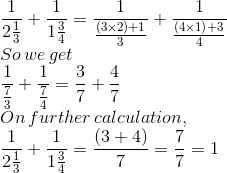9. If 1/3 + 1/2 + 1/x = 4, then x = ?
(a) 5/18
(b) 6/19
(c) 18/5
(d) 24/11

Solution:

The option (b) is correct answer.

It is given that

1/3 + ½ + 1/x = 4

On further calculation

1/x = 4 – 1/3 – 1/2

By taking LCM of 3 and 2 as 6

1/x = 24/6 – 2/6 – 3/6

So we get

1/x = (24 – 2 – 3)/ 6 = 19/6

Hence, x = 6/19

10. If 1/2 + 1/x = 2, then x =
(a) 2/5
(b) 5/2
(c) 3/2
(d) 2/3

Solution:

The option (d) is correct answer.

It is given that

½ + 1/x = 2

On further calculation

1/x = 2 – 1/2

By taking LCM as 2 we get

1/x = 4/2 – 1/2 = (4 – 1)/2 = 3/2

Hence, x = 2/3

11. Which of the following fractions is the smallest?
1/2,3/7,3/5,4/9
(a) 4/9
(b) 3/5
(c) 3/7
(d) 1/2

Solution:

The option (c) is correct answer.

We know that the LCM of numerator is 12

By converting each fraction to an equivalent fraction having 12 as numerator

1/2 = 1/2 × 12/12 = 12/24

3/7 = 3/7 × 4/4 = 12/28

3/5 = 3/5 × 4/4 = 12/20

4/9 = 4/9 × 3/3 = 12/27

We know that if the numerator is same the fraction having larger denominator is the smallest.

Hence, 3/7 is the smallest fraction.

12. Which of the following fractions is the greatest of all?

7/8, 6/7, 4/5, 5/6
(a) 6/7
(b) 4/5
(c) 5/6
(d) 7/8

Solution:

The option (d) is correct answer.

We know that the LCM of 8, 7, 6 and 5 is 840

By converting each fraction to an equivalent fraction having 840 as denominator

7/8 = 7/8 × 105/105 = 735/840

6/7 = 6/7 × 120/120 = 720/840

4/5 = 4/5 × 168/168 = 672/840

5/6 = 5/6 × 140/140 = 700/840

We know that if the denominator is same the fraction having larger numerator is the greatest.

Hence, 7/8 is the greatest fraction.

13. What is the value of a+b/ a−b, If a/b=4?
(a) 3/5
(b) 5/3
(c) 4/5
(d) 5/4

Solution:

The option (b) is correct answer.

It is given that a/b = 4

We can write it as a = 4b

By substituting the value of a in a+b/a-b

a+b/a-b = 4b+b/4b-b = 5b/3b

Dividing numerator and denominator by b, the value is 5/3.

14. If a/b = 4/3, then the value of 6a+4b/ 6a-5b is
(a) −1
(b) 3
(c) 4
(d) 5

Solution:

The option (c) is correct answer.

It is given that a/b = 4/3

We can write it as a = 4b/3

By substituting the value of a in 6a+4b/6a-5b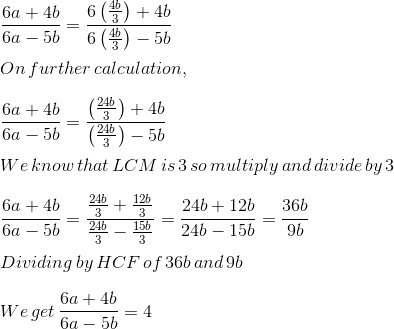15. If 1/5 – 1/6 = 4/x, then x =
(a) −120
(b) −100
(c) 100
(d) 120

Solution:

The option (d) is correct answer.

It is given that

1/5 – 1/6 = 4/x

LCM of 5 and 6 is 30

4/x = 6/30 – 5/30

On further calculation

4/x = 1/30

So we get

x = 4 (30) = 120

16. The fraction to be added to 6 7/15 to get 8 1/5 is equal to
(a) 11/15
(b) 1 1/15
(c) 44/3
(d) 3/44

Solution:

The option (b) is correct answer.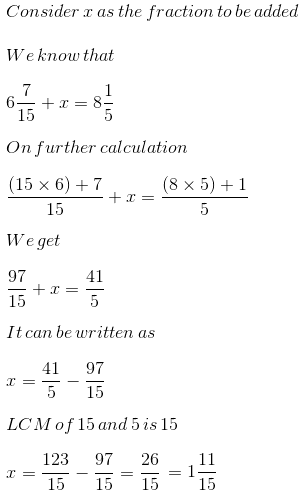17. If 45/60 is equivalent to 3/x, then x =
(a) 5
(b) 4
(c) 6
(d) 20

Solution:

The option (b) is correct answer.

It is given that

45/60 = 3/x

By cross multiplication

45 × x = 3 × 60

It can be written as

x = (3 × 60)/45 = 180/45

Dividing the fraction by HCF

(180 ÷ 45)/(45÷45) = 4

18. A fraction equivalent to 45/105 is
(a) 6/14
(b) 4/7
(c) 5/7
(d) 7/5

Solution:

The option (a) is correct answer.

The given fraction is 45/105

By dividing the numerator and denominator with the HCF

(45 ÷ 15)/(105÷15) = 3/7

On further calculation

3/7 = 3/7 × 2/2 = 6/14

19. 5/8 + 3/4 – 7/12 is equal to
(a) 15/24
(b) 17/24
(c) 19/24
(d) 21/24

Solution:

The option (c) is correct answer.

The given fraction is

5/8 + 3/4 – 7/12

We know that the LCM is 24

= (5 × 3)/ (8 × 3) + (3 × 6)/ (4 × 6) – (7 × 2)/ (12 × 2)

On further calculation

= 15/24 + 18/24 – 14/24

So we get

= 19/24

20. The correct fraction in the box □ is □ – 5/8=1/4
(a) 6/8
(b) 7/8
(c) 1/2
(d) None of these

Solution:

The option (b) is correct answer.

The given equation is

□ – 5/8 = 1/4

It can be written as

□ = 1/4 + 5/8

We know that the LCM is 8

□ = 2/8 + 5/8 = 7/8